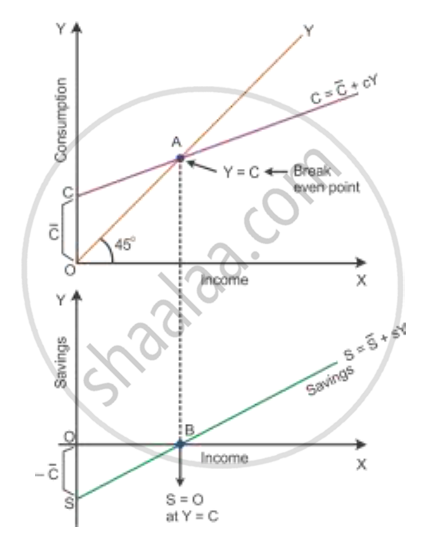Share

# Given Saving Curve, Derive Consumption Curve and State the Steps in Doing So. Use Diagram. - CBSE (Arts) Class 12 - Economics

#### Questions

Given saving curve, derive consumption curve and state the steps in doing so. Use diagram.

Outline the steps required to be taken in deriving the Consumption Curve from the given Saving Curve. Use Diagram.

#### Solution

In the diagram, the supply curve is given as the SS curve and -barC  represents negative savings. At the breakeven Point B, we find that Y = C and S = O.

Derivation of the consumption curve from the saving curve:-

Given the SS curve, let us consider OS = OC. At Point B, draw a perpendicular 45° line towards Point A. Points C and A are joined to produce a straight line upward sloping consumption curve CC.Is there an error in this question or solution?

#### Video TutorialsVIEW ALL 

Solution Given Saving Curve, Derive Consumption Curve and State the Steps in Doing So. Use Diagram. Concept: Supply Curve and Schedule.
S